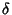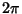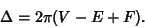## Descartes Total Angular Defect

The total angular defect is the sum of the Angular Defects over all Vertices of a Polyhedron, where the Angular Defectat a given Vertex is the difference between the sum of face angles and. For any convex Polyhedron, the Descartes total angular defect is(1)

This is equivalent to the Polyhedral Formula for a closed rectilinear surface, which satisfies(2)

A Polyhedron withequivalent Vertices is called a Platonic Solid and can be assigned a Schläfli Symbol. It then satisfies(3)

and(4)

so(5)